# Weyl correspondence

(diff) ← Older revision | Latest revision (diff) | Newer revision → (diff)

A mapping between a class of (generalized) functions on the phase space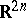and the set of closed densely defined operators on the Hilbert space[a1] (cf. also Generalized function; Hilbert space). It is defined as follows: Letbe an arbitrary point of(called phase space) and let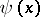be an arbitrary vector on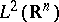. For a point in, the Grossmann–Royer operatoris defined as [a2], [a3]: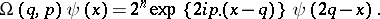Now, take a function. The Weyl mappingis defined as [a4], [a5]:The Weyl mapping defines the Weyl correspondence between functions and operators. It has the following properties:

i) It is linear and one-to-one.

ii) Ifis bounded, the operator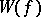is also bounded.

iii) Ifis real,is self-adjoint (cf. also Self-adjoint operator).

iv) Let, the Schwartz space, and define the Weyl product as [a6]: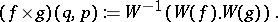The Weyl product defines an algebra structure on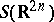, which admits a closure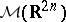with the topology of the space of tempered distributions,(cf. also Generalized functions, space of). The algebraincludes the space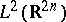and the Weyl mapping can be uniquely extended to.

v) Obviously,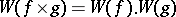.

vi) Ifis the multiplication operator onand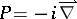, then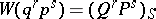, wheredenotes the symmetric product offactorsandfactors.

vii) For any positive trace-class operatoron, there exists a signed measure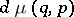on, such that for any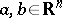,This measure has a Radon–Nikodým derivative (cf. also Radon–Nikodým theorem) with respect to the Lebesgue measure, which is called the Wigner function associated to.

The Weyl correspondence is used by physicists to formulate quantum mechanics of non-relativistic systems without spin or other constraints on the flat phase space[a5].

The Stratonovich–Weyl correspondence [a4], [a5], [a7] or Stratonovich–Weyl mapping generalizes the Weyl mapping to other types of phase spaces. Choose a co-adjoint orbitof the representation group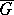of a certain Lie groupof symmetries of a given physical system as phase space. The Hilbert space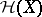used here supports a linear unitary irreducible representation of the groupassociated to. Then, a generalization of the Grossmann–Royer operator is needed, associating each pointof the orbitwith a self-adjoint operator. Then, for a suitable class of measurable functionson, one defines: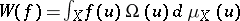, where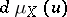is a measure onthat is invariant under the action of; such a measure is uniquely defined, up to a multiplicative constant.

The Weyl correspondence is a particular case of the Stratonovich–Weyl correspondence for whichis the Heisenberg group, [a1], [a5].

How to Cite This Entry:
Weyl correspondence. Encyclopedia of Mathematics. URL: http://encyclopediaofmath.org/index.php?title=Weyl_correspondence&oldid=18852
This article was adapted from an original article by M. Gadella (originator), which appeared in Encyclopedia of Mathematics - ISBN 1402006098. See original article